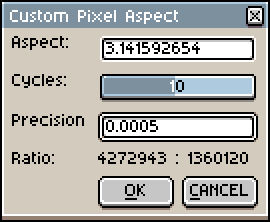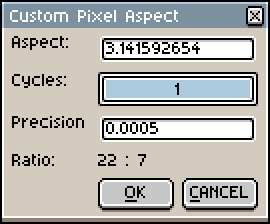# Custom pixel aspect ratio

Hey there! I love Aseprite and it’s my go to choice for anything pixel art related. Except now I’m working on a game for a system that uses a 5:4 aspect ratio!

I started this topic because I could not find anything resembling a roadmap that I could check to see if this is a planned feature. I would very much like to have custom pixel aspect ratio. Anyone working on retro systems should encounter similar problems.

For now I’ll just have to…use gimp. I hate gimp. Please, set me free from Gimp, elders of aseprite. I can sacrifice a goat. Well, not a goat, I like goats. I’ll leave you a fruit basket or something. Near a volcano.

1 Like

Hey there! I played with Aseprite’s data files for a little bit and it turns out that you can actually import your own custom ratios by editing a text file. Take a look!

New sprite window

Sprite properties. Those pixels certainly don’t look a 1:1 pixel to me!

You have to edit 2 files to show the custom ratio in sprite properties and the new sprite window.

Keep in mind that this might cause some bugs since I don’t if we should even do this. Only @dacap will be able to let us know whether it’s fine for us to mess around with the internal settings. But looking at the structure of the config files, it looks like it’s designed to do this kind of thing.

2 Likes

Ooh! I am willing to take some risks. Thanks for the info, haloflooder!

I am free from gimp for now.

edit: Yup. Tested and it seems to be working fine for now. Just wanted to thank you again for taking the trouble.

1 Like

@haloflooder hi there! no problem to mess with the xml files, the only problem is that in a next update your changes will be overwritten; anyway several times ago I gave the same advice for custom pixel aspect ratios (modifying the xml files manually)I hope to have a better way in a near futureHi there! Sorry to take this thread back to life. Do you guys know if it’s possible to use decimals in the aspect ratio? I need to use (0.8*1) I tried with modifying the files with “0.8:1” and “0,8:1” and I got nothing. Don’t know if it’s aseprite doesn’t allowing to use decimals in the aspect ratio or if it’s me don’t knowing how to write them in codeUnless I’m misunderstanding, a 0.8:1 aspect ratio would be the same as 4:5

1 Like

Welcome to the forum!

I was curious as to whether a decimal input was possible or practical. To give you a demo of why it’s not practical in my opinion, I looked up a how to convert a decimal to a fraction. I made a Lua script based on that:It’s doable if you use it carefully… But as you can imagine, it’s faster, easier and more reliable to calculate, say, `1.77778` from `16 : 9` than the reverse. In general, software designers and programmers have to develop defensively.

[Edit: If you restyle the while loop to stop if either the numerator or the denominator grow beyond a limit, like 16… Then maybe my opinion changes and this has more promise. Either way, it was fun to think about and test.]

``````-- Stack Overflow solution by onyambu
-- https://stackoverflow.com/a/64828741
local function toRatio(number, cycles, precision)
local cVerif = cycles or 10
local pVerif = precision or 5e-4
cVerif = math.max(1, math.abs(cycles))
pVerif = math.max(1e-10, math.abs(pVerif))

local sgnNum = 0
if number > 0 then sgnNum = 1 end
if number < 0 then sgnNum = -1 end
if number == 0 then return 0, 0 end

local absNum = math.abs(number)
local truncNum =  math.tointeger(absNum)
local fracNum = absNum - truncNum

local aVec = { truncNum, 1 }
local bVec = { 1, 0 }

local counter = 0
while fracNum > pVerif and counter < cVerif do
local newNum = 1.0 / fracNum
local integral = math.tointeger(newNum)

local temp = aVec
aVec = { integral * aVec + bVec,
integral * aVec + bVec }
bVec = temp

fracNum = newNum - integral
counter = counter + 1
end

return sgnNum * aVec, aVec
end

local dlg = Dialog { title = "Custom Pixel Aspect" }

local function updateLabel()
local args = dlg.data
local aspectRatio = args.aspectRatio
local cycles = args.cycles
local precision = args.precision
local ant, cons = toRatio(aspectRatio, cycles, precision)
local str = string.format("%d : %d", ant, cons)
dlg:modify { id = "decLabel", text = str }
end

dlg:number {
id = "aspectRatio",
label = "Aspect:",
text = "1.0",
decimals = 5,
onchange = updateLabel
}

dlg:slider {
id = "cycles",
label = "Cycles:",
value = 10,
min = 1,
max = 20,
onchange = updateLabel
}

dlg:number {
id = "precision",
label = "Precision",
text = string.format("%.8f", 5e-4),
decimals = 6,
onchange = updateLabel
}

dlg:newrow { always = false }

dlg:label {
id = "decLabel",
label = "Ratio:",
text = "1:1"
}

dlg:newrow { always = false }

dlg:button {
id = "confirm",
text = "&OK",
focus = false,
onclick = function()
local activeSprite = app.activeSprite
if not activeSprite then return end
local args = dlg.data
local aspectRatio = args.aspectRatio
local precision = args.precision
local cycles = args.cycles
local ant, cons = toRatio(aspectRatio, cycles, precision)

if ant == 0 then return end
if cons == 0 then return end

ant = math.abs(ant)
cons = math.abs(cons)

-- There should be some reasonable stopping point...
local stopPoint = 16
if ant >= stopPoint or cons >= stopPoint then
return
end

-- Do not assign Size(0, 0) or a size with either
-- negative width or negative height to pixel ratio.
activeSprite.pixelRatio = Size(ant, cons)
end
}

dlg:button {
id = "cancel",
text = "&CANCEL",
focus = true,
onclick = function()
dlg:close()
end
}

dlg:show { wait = false }
``````

(Custom aspect ratios done by script are not picked up by the new sprite or sprite properties dialog, which is an advantage of the XML.)

Cheers,
Jeremy